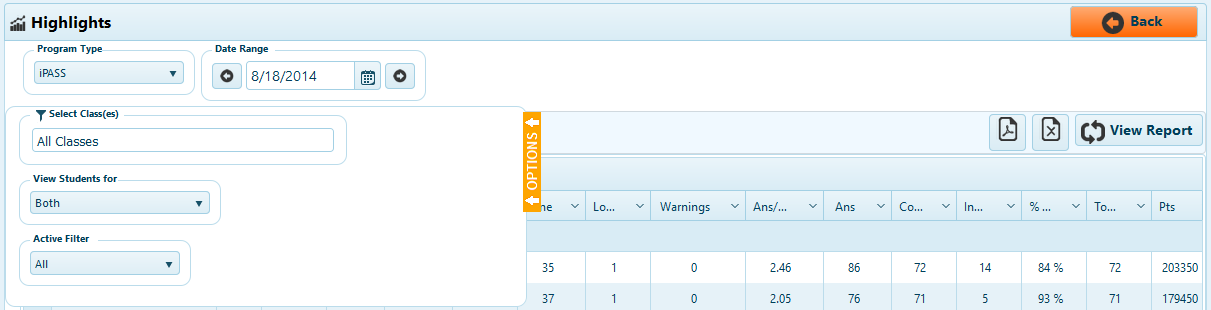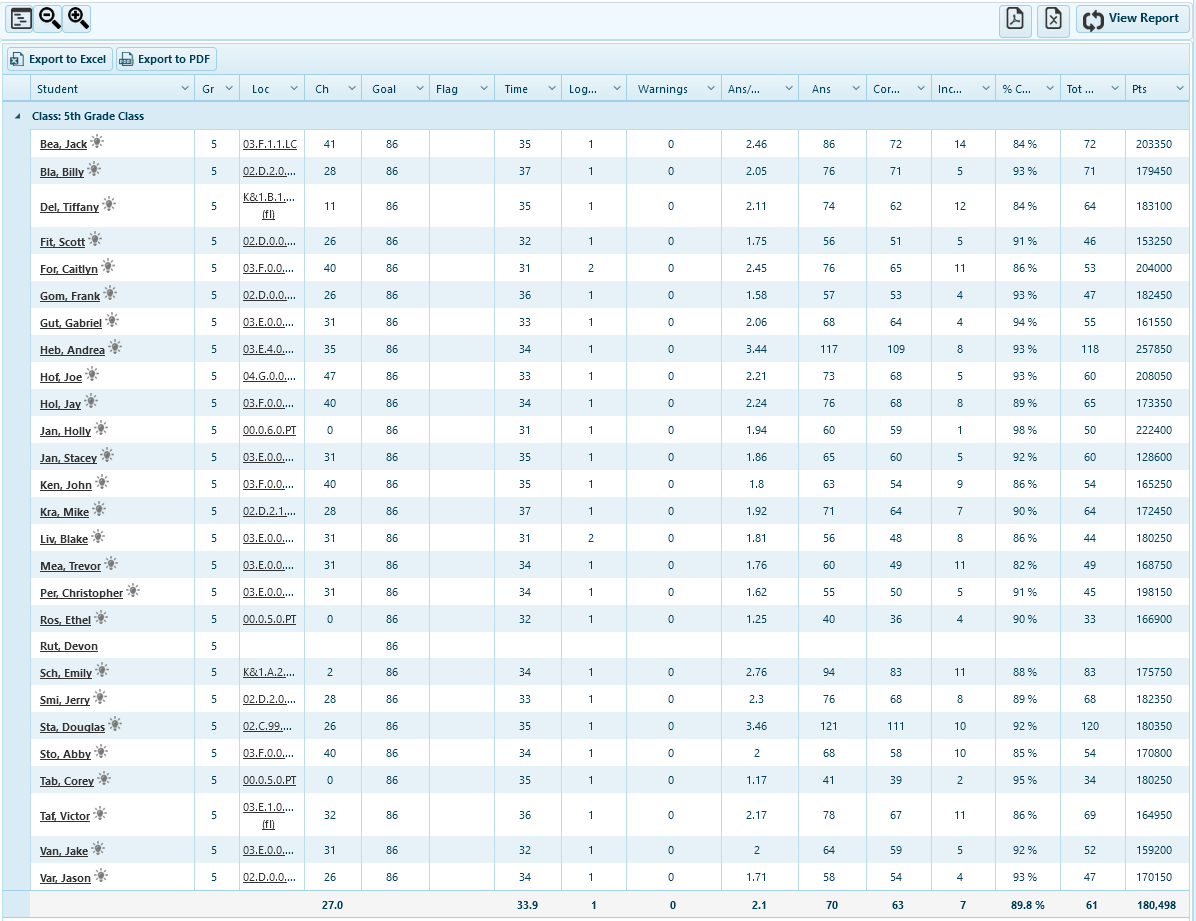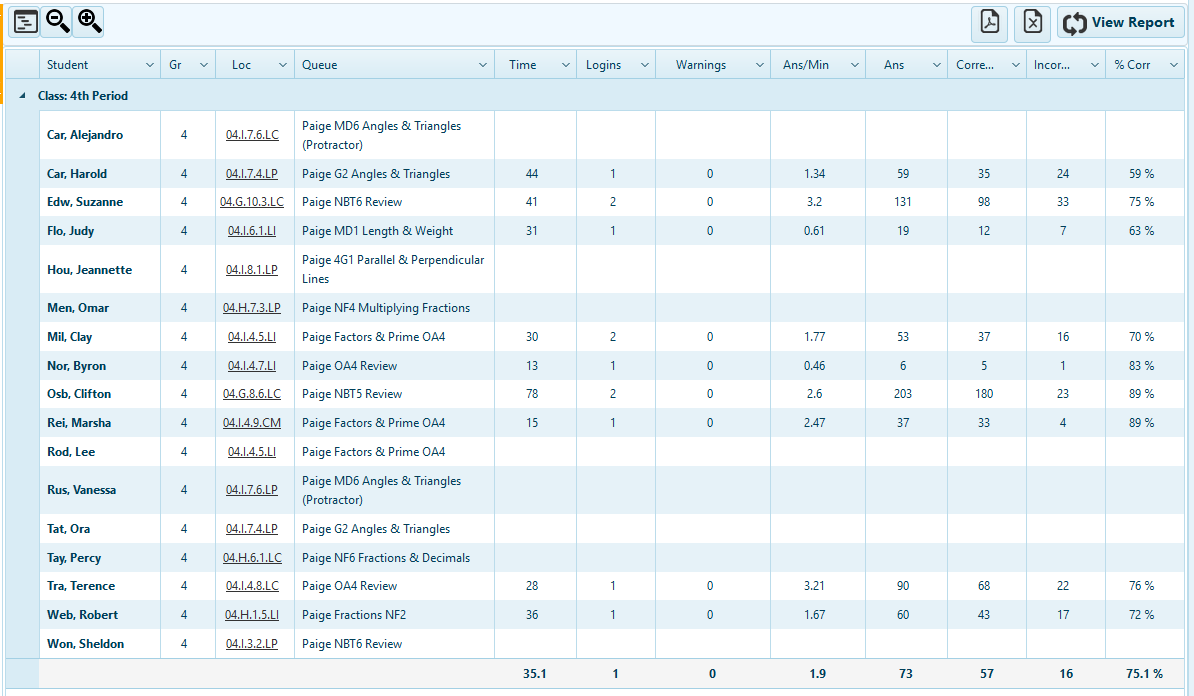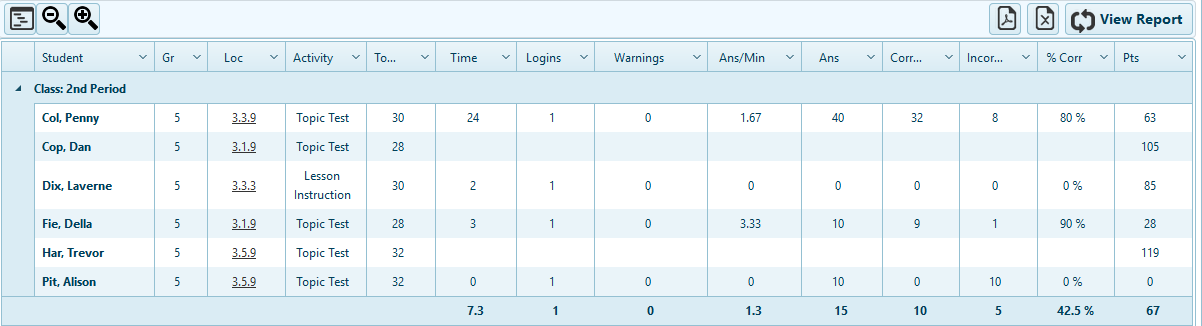# Highlights

### The Highlights report is available at the teacher level only. It enables teachers to monitor student activity in real time.The Highlights report displays by program, and all five programs have a similar Highlights report.

1. Program Type - Choose the program to view activity for the selected day.
2. Date - Select a specific day to view. The default is the current day, but previous days can be selected.
3. Options - Alter the options to change what is viewed in the report.
• Select Class(es) - View all classes, multiple classes, or view just one class.
• View Students for - Choose to view students for Teacher of Record, Virtual View, or both (the default).
• Active Filter - Choose to view only students with activity on the day selected, only students with no activity, or all students (the default).
4. Export - Export to PDF or Excel.The iLearn Math Highlights report displays the following, for the day selected unless otherwise indicated:

1. Student - Name of the student
2. Gr - The student's enrolled grade level
3. Location - The current location in iLearn Math
4. Chap # - The current chapter number in a numeric sequence from the first chapter to the last chapter in iLearn Math
5. Goal - The chapter number in the sequence representing the last chapter in a student's grade level
6. Flag - Progress Flag in iLearn Math if applicable - blank if the student has no Progress Flag
7. Time - The time in minutes of activity in iLearn Math
8. # Logins - The number of times the student logged into iLearn Math
9. Logout Warnings - The number of logout warnings the student received in iLearn Math
10. Ans/Min - The number of answers per minutes the student entered in iLearn Math
11. Tot Ans - The total number of answers the student entered in iLearn Math
12. Correct Ans - The total number of correct answers the student submitted in iLearn Math
13. % Correct - The percent correct answers entered by the student in iLearn Math
14. Total Points - The student's total number of correct answers in iLearn Math to dateThe Assignments Highlights report displays the following, for the day selected unless otherwise indicated:

1. Student - Name of the student
3. Location - The current location of the student
4. Queue - The name of the queue of current student activity
5. Time - The time in minutes of activity in Assignments
6. # Logins - The number of times the student logged into Assignments
7. Logout Warnings - The number of logout warnings the student received in Assignments
8. Ans/Min - The number of answers the student entered in Assignments
9. Total Ans - The total number of answers the student entered in Assignments
10. Correct Ans - The total number of correct answers the student entered in Assignments
11. % Correct - The percent correct answers entered by the student in AssignmentsThe Assessments Highlights report displays the following, for the day selected unless otherwise indicated:

1. Student - Name of student
2. Gr - The student's enrolled grade level
3. Assessment - The assessment of current student activity
4. Type - The type of assessment
5. Test Gr - The grade level of test the student is currently in
6. Time(Min) - The time in minutes of student activity
7. # Logins - The number of times the student logged in
8. Logout Warnings - The number of logout warnings the student received
9. Ans/Min - The number of answers the student entered
10. Total Ans - The total number of answers the student entered
11. Correct Ans - The total number of correct answers the student entered
12. % Correct - The percent correct answers entered by the studentThe vBook Highlights report displays the following, for the day selected unless otherwise indicated:

1. Student - Name of the student
2. Gr - The student's enrolled grade level
3. Location - The location of latest activity in vBook
4. Topic # - The number in sequence of the topics in vBook, for the latest activity
5. Activity - The latest type of activity in vBook
6. Time(Min) - The time in minutes of student activity in vBook
7. # Logins - The number of times the student logged into vBook
8. An per Min - The number of answers the student entered in vBook
9. Total Ans - The total number of answers the student entered in vBook
10. Correct Ans - The total number of correct answers the student entered in vBook
11. % Correct - The percent correct answers entered by the student in vBook
12. Total Points - The total number of correct answers in vBook to date

Use the Highlights report while students are in class to monitor the program students are in, and to view quick measures on student performance.

`Calendly.initBadgeWidget({url: 'https://calendly.com/ilearnteam', text: 'Register for Training Session', color: '#00a2ff', branding: false});`